# ITEM PLACING CODE: Place custom books (or items) from outside loaded mods.

## Recommended Posts

This is not a request.  Instead, this is a post supplying actual working code.  And what for?  This allows a modder to bring items (basicallly books) from another mod and be able to place it in visibly in the world.  And this, without relying on being dependent upon the mod supplying the item.

Confused?  I bet.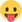Let's say you are making a mod like.. um... The Underking.  He's a character from as far back as Daggerfall.  Now let's say you wanted to have a number of books here and there, scattered about and on shelves, but didn't feel like copying the Daggerfall Books mod I made.  And you wanted the books as optional.  With the scripts, you could place a book on a shelf by code with this command:

Call BookishPlace270 StudyShelf1Ref, "Books of Daggerfall.esp", "000F1C", -4.568, -17.0, 55.449, 10.0

The above code would grab from the Daggerfall book mod a book with the FormID of "xx000F1C", that book being "A Scholar's Guide to Nymphs", and place it on a bookshelf with the Reference ID of "StudyShelf1Ref".  The X Y and Z locations are relative to the center of the Bookshelf and the book is given a 10 degree tilt.

The code would look the make sure the Daggerfall books mod was loaded, otherwise it would exit peacefully.  And it would place it on the designated bookshelf it it existed, complete with the mod-specified jacket cover.

There are FOUR sets of scripts, each for a bookshelf at a specific angle:  0 degrees, 90 degrees, 180 degrees and 270 degrees.  This would make it a bit easier to handle (I would figure).  And the code assumes that you want the jacket cover art to be rendered, and facing the player rather than showing the book spine.

0 Degrees

```
scn BookishPlace0

;; Book placing on shelf with books that can be tilted. Shelf at 0 rotation

ref ShelfRef        ; Param: Shelf at 0 degree angle
string_var modname  ; Param: Mod carrying book
string_var formid   ; Param: Book Form ID to load
float xPos          ; Param: x-Position
float yPos          ; Param: y-Position
float zPos          ; Param: z-Position
float Tilt          ; Param: Tilt Angle

ref BookRef         ; Generated Reference object
float posx          ; Shelf x-position for calculation
float posy          ; Shelf y-position for calculation
float posz          ; Shelf z-position for calculation
float angletilt     ; Tilt Angle Calculation value

Begin Function { ShelfRef modname formid xPos yPos zPos, Tilt }

;; Exit if mod is not loaded
if ( IsModLoaded \$modname ) == 0
return
endif

;; Acquire book and place at shelf
Let BookRef := GetFormFromMod \$modname \$formid
Let BookRef := ShelfRef.PlaceAtMe BookRef 1 0 0

;; Acquire shelf coords
Let posx := ShelfRef.GetPos x
Let posy := ShelfRef.GetPos y
Let posz := ShelfRef.GetPos z

;; Calculate coordinates
Let posx += xPos
Let posy += yPos
Let posz -= zPos

;; Move book based on coords
BookRef.SetPos x, posx
BookRef.SetPos y, posy
BookRef.SetPos z, posz

;; Upright Spine if -360 tilt
if tilt == -360
BookRef.SetAngle x, 270
BookRef.SetAngle y, 270
BookRef.SetAngle z, 0
return
endif

;; Calculate angle
set angletilt to 90.0
set angletilt to angletilt + Tilt

BookRef.SetAngle x, 90.0
BookRef.SetAngle y, 180.0
BookRef.SetAngle z, 180.0

end

```

90 Degrees

```
scn  BookishPlace90

;; Book placing on shelf with books that can be tilted. Shelf at 90 rotation

ref ShelfRef        ; Param: Shelf at 90 degree angle
string_var modname  ; Param: Mod carrying book
string_var formid   ; Param: Book Form ID to load
float xPos          ; Param: x-Position
float yPos          ; Param: y-Position
float zPos          ; Param: z-Position
float Tilt          ; Param: Tilt Angle

ref BookRef         ; Generated Reference object
float posx          ; Shelf x-position for calculation
float posy          ; Shelf y-position for calculation
float posz          ; Shelf z-position for calculation
float angletilt     ; Tilt Angle Calculation value

Begin Function { ShelfRef modname formid xPos yPos zPos, Tilt }

;; Exit if mod is not loaded
if ( IsModLoaded \$modname ) == 0
return
endif

;; Acquire book and place at shelf
let BookRef := GetFormFromMod \$modname \$formid
let BookRef := ShelfRef.PlaceAtMe BookRef 1 0 0

;; Acquire shelf coords
let posx := ShelfRef.GetPos x
let posy := ShelfRef.GetPos y
let posz := ShelfRef.GetPos z

;; Calculate coordinates
let posx += xPos
let posY += yPos
let posZ -= zPos

;; Move book based on coords
BookRef.SetPos x, posx
BookRef.SetPos y, posy
BookRef.SetPos z, posZ

;; Upright Spine if -360 tilt
if tilt == -360
BookRef.SetAngle x, 270
BookRef.SetAngle y, 0
BookRef.SetAngle z, 0
return
endif

;; Calculate angle
set angletilt to 90.0
set angletilt to angletilt + Tilt

BookRef.SetAngle x, 180.0
BookRef.SetAngle y, angletilt
BookRef.SetAngle z, 270.0

end

```

180 Degrees

```
scn  BookishPlace180

;; Book placing on shelf with books that can be tilted. Shelf at 180 rotation

ref ShelfRef        ; Param: Shelf at 180 degree angle
string_var modname  ; Param: Mod carrying book
string_var formid   ; Param: Book Form ID to load
float xPos          ; Param: x-Position
float yPos          ; Param: y-Position
float zPos          ; Param: z-Position
float Tilt          ; Param: Tilt Angle

ref BookRef         ; Generated Reference object
float posx          ; Shelf x-position for calculation
float posy          ; Shelf y-position for calculation
float posz          ; Shelf z-position for calculation
float angletilt     ; Tilt Angle Calculation value

Begin Function { ShelfRef modname formid xPos yPos zPos, Tilt }

;; Exit if mod is not loaded
if ( IsModLoaded \$modname ) == 0
return
endif

;; Acquire book and place at shelf
Let BookRef := GetFormFromMod \$modname \$formid
Let BookRef := ShelfRef.PlaceAtMe BookRef 1 0 0

;; Acquire shelf coords
Let posx := ShelfRef.GetPos x
Let posy := ShelfRef.GetPos y
Let posz := ShelfRef.GetPos z

;; Calculate coordinates
Let posx += xPos
Let posy += yPos
Let posz -= zPos

;; Move book based on coords
BookRef.SetPos x, posx
BookRef.SetPos y, posy
BookRef.SetPos z, posz

;; Upright Spine if -360 tilt
if tilt == -360
BookRef.SetAngle x, 270
BookRef.SetAngle y, 90
BookRef.SetAngle z, 0
return
endif

;; Calculate angle
set angletilt to 90.0
set angletilt to angletilt - Tilt

BookRef.SetAngle x, 90.0
BookRef.SetAngle y, 0.0
BookRef.SetAngle z, 180.0

end

```

270 Degrees

```
scn  BookishPlace270

;; Book placing on shelf with books that can be tilted. Shelf at 270 rotation

ref ShelfRef        ; Param: Shelf at 270 degree angle
string_var modname  ; Param: Mod carrying book
string_var formid   ; Param: Book Form ID to load
float xPos          ; Param: x-Position
float yPos          ; Param: y-Position
float zPos          ; Param: z-Position
float Tilt          ; Param: Tilt Angle

ref BookRef         ; Generated Reference object
float posx          ; Shelf x-position for calculation
float posy          ; Shelf y-position for calculation
float posz          ; Shelf z-position for calculation
float angletilt     ; Tilt Angle Calculation value

Begin Function { ShelfRef modname formid xPos yPos zPos, Tilt }

;; Exit if mod is not loaded
if ( IsModLoaded \$modname ) == 0
return
endif

;; Acquire book and place at shelf
let BookRef := GetFormFromMod \$modname \$formid
let BookRef := ShelfRef.PlaceAtMe BookRef 1 0 0

;; Acquire shelf coords
let posx := ShelfRef.GetPos x
let posy := ShelfRef.GetPos y
let posz := ShelfRef.GetPos z

;; Calculate coordinates
let posx += xPos
let posY += yPos
let posZ -= zPos

;; Move book based on coords
BookRef.SetPos x, posx
BookRef.SetPos y, posy
BookRef.SetPos z, posZ

;; Upright Spine if -360 tilt
if tilt == -360
BookRef.SetAngle x, 90
BookRef.SetAngle y, 0
BookRef.SetAngle z, 180
return
endif

;; Calculate angle
set angletilt to 270
set angletilt to angletilt - Tilt

BookRef.SetAngle x, 180.0
BookRef.SetAngle y, angletilt
BookRef.SetAngle z, 90.0

end

```

Each script uses the same basic Syntax

Call "SCRIPT"  ShelfReferenceModNameFormID X-Pos, Y-Pos, Z-Pos, Tilt

• "SCRIPT"              One of the above four scripts
• ShelfReference:    The bookshelf (or like) which must be a Persistent Reference
• ModName:          The name of the mod where the item originates
• FormID:               The FormID of the individual item or book
• X/Y/Z-Pos:           The X-Pos, Y-Pos and Z-Pos indicates where the item is placed relative to the bookshelf
• Tilt*:                      This is the tilt forward/backward of the book.  0 Tilt has it straight up.*

* This was designed to have books placed on bookshelves where the jacket covers are facing the player.  But if the TILT value (above) is set to -360, then the book will be placed with the book spine facing the player as most vanilla bookshelves do.

Book jacket covers are assumed to be made like those within the spoilers

Folio (Large Tome) Works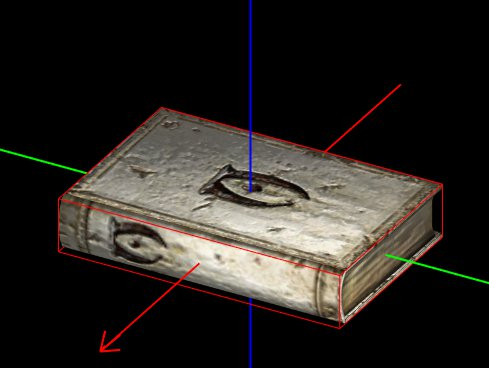As you can see below, the textures are not reversed or flipped.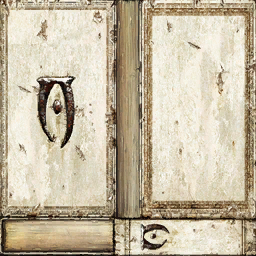Quarto (midsize) Works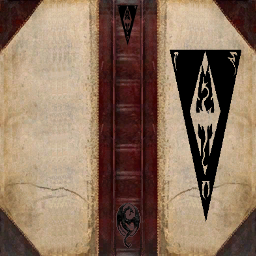Used for my Daggerfall books, the text and graphic art for the spine is horizontally flipped.

Octavo (Small-Sized) Works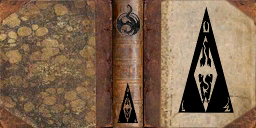This too was used for my Daggerfall books, the cover art vertically flipped while the spine a 180 rotation.

What if you turned "000F1C"   into a leveled list of books , that would create a bunch on books looped into each other right, lol.

That, I never tested.  In that case it may choose a random book from the leveled list of books.

20 minutes ago, LongDukDong said:

That, I never tested.  In that case it may choose a random book from the leveled list of books.

But as we both know you can set the count of a leveled list within a leveled list.

but won't such copies clog the save?

What I mean is to make a lot of copies, but for a while so that they are not, after the game does not restart. Or is there a way to destroy copies from the memory of the game and the save (so that there is no overflow of generated objects)

One would consider that you would want to only load the file(s) once.  I mean, have it appear in a startup quest. And once the mod is actually detected... run them and add... and be done with it.

short primabookone

Begin GamePlay

if primabookone == 0

call BookishPlace90 StudyShelf1Ref, "LoversPrimaBooks1.esp", "000ED3", -52.568, -17.0, 55.449, 10.0

call BookishPlace90 StudyShelf1Ref, "LoversPrimaBooks1.esp", "000ED4", -28.568, -17.0, 55.449, 10.0

call BookishPlace90 StudyShelf1Ref, "LoversPrimaBooks1.esp", "000ED5"-4.568, -17.0, 55.449, 10.0

call BookishPlace90 StudyShelf1Ref, "LoversPrimaBooks1.esp", "000ED6"24.568, -17.0, 55.449, 10.0

call BookishPlace90 StudyShelf1Ref, "LoversPrimaBooks1.esp", "000ED7"46.568, -17.0, 55.449, 10.0

set primabookone to 1

endif

End

Trust me, I ran into some hilarity when I was working on the code.  At one point, I mistook the code that set the number of 'items' as the x position and set it to drop 4000 items on a shelf.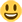It was like Hermaeous Mora's realm of Black Books!!!!Some thought went into how this works.

## Create an account

Register a new account